Publicité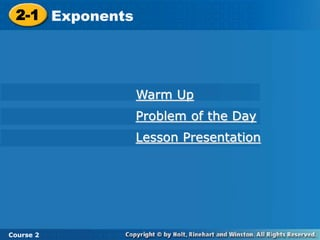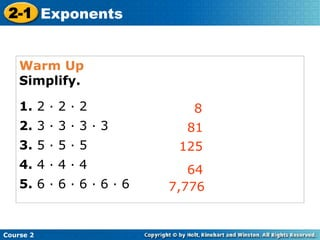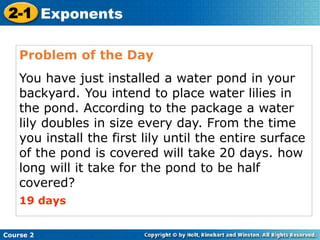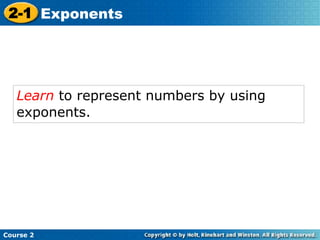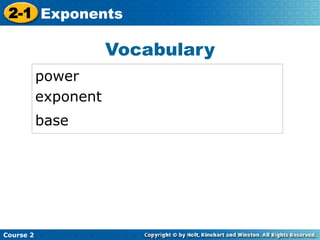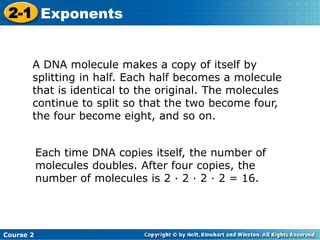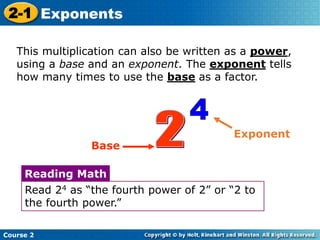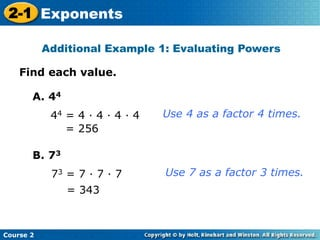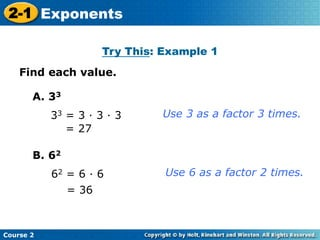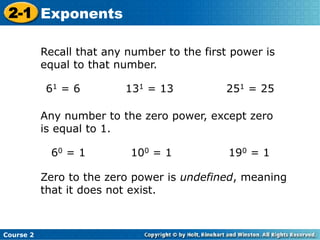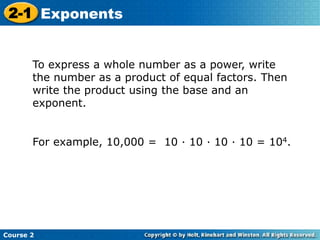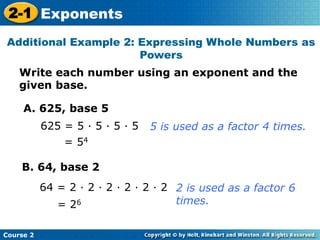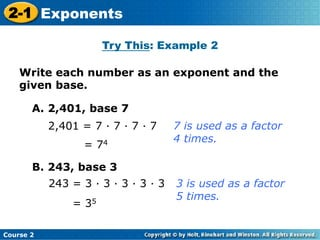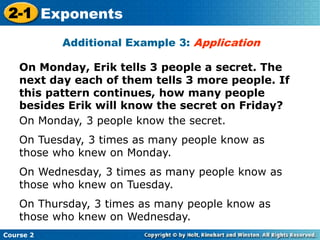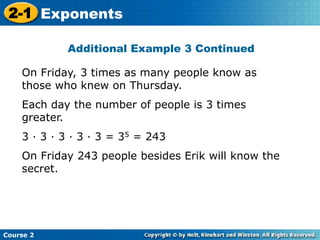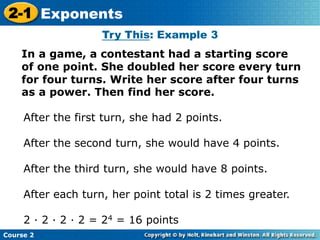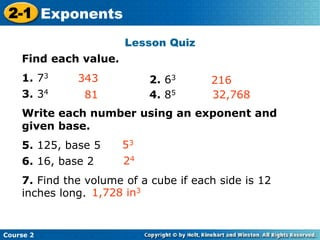1 sur 17
Publicité

### Exponents

1. 2-1 Exponents Course 2 Warm Up Problem of the Day Lesson Presentation
2. Warm Up Simplify. 1. 2 · 2 · 2 2. 3 · 3 · 3 · 3 3. 5 · 5 · 5 4. 4 · 4 · 4 5. 6 · 6 · 6 · 6 · 6 8 81 125 Course 2 2-1 Exponents 64 7,776
3. Problem of the Day You have just installed a water pond in your backyard. You intend to place water lilies in the pond. According to the package a water lily doubles in size every day. From the time you install the first lily until the entire surface of the pond is covered will take 20 days. how long will it take for the pond to be half covered? 19 days Course 2 2-1 Exponents
4. Learn to represent numbers by using exponents. Course 2 2-1 Exponents
5. Vocabulary power exponent base Insert Lesson Title Here Course 2 2-1 Exponents
6. Course 2 2-1 Exponents A DNA molecule makes a copy of itself by splitting in half. Each half becomes a molecule that is identical to the original. The molecules continue to split so that the two become four, the four become eight, and so on. Each time DNA copies itself, the number of molecules doubles. After four copies, the number of molecules is 2 · 2 · 2 · 2 = 16.
7. Course 2 2-1 Exponents This multiplication can also be written as a power, using a base and an exponent. The exponent tells how many times to use the base as a factor. Base Exponent Read 24 as “the fourth power of 2” or “2 to the fourth power.” Reading Math
8. Find each value. Additional Example 1: Evaluating Powers Course 2 2-1 Exponents A. 44 44 = 4 · 4 · 4 · 4 = 256 B. 73 73 = 7 · 7 · 7 = 343 Use 4 as a factor 4 times. Use 7 as a factor 3 times.
9. Try This: Example 1 Insert Lesson Title Here Course 2 2-1 Exponents Find each value. A. 33 33 = 3 · 3 · 3 = 27 B. 62 62 = 6 · 6 = 36 Use 3 as a factor 3 times. Use 6 as a factor 2 times.
10. Course 2 2-1 Exponents Recall that any number to the first power is equal to that number. 61 = 6 131 = 13 251 = 25 Any number to the zero power, except zero is equal to 1. 60 = 1 100 = 1 190 = 1 Zero to the zero power is undefined, meaning that it does not exist.
11. Course 2 2-1 Exponents To express a whole number as a power, write the number as a product of equal factors. Then write the product using the base and an exponent. For example, 10,000 = 10 · 10 · 10 · 10 = 104.
12. Write each number using an exponent and the given base. Additional Example 2: Expressing Whole Numbers as Powers Course 2 2-1 Exponents A. 625, base 5 625 = 5 · 5 · 5 · 5 = 54 5 is used as a factor 4 times. B. 64, base 2 64 = 2 · 2 · 2 · 2 · 2 · 2 = 26 2 is used as a factor 6 times.
13. Try This: Example 2 Insert Lesson Title Here Course 2 2-1 Exponents Write each number as an exponent and the given base. A. 2,401, base 7 2,401 = 7 · 7 · 7 · 7 = 74 7 is used as a factor 4 times. B. 243, base 3 243 = 3 · 3 · 3 · 3 · 3 = 35 3 is used as a factor 5 times.
14. On Monday, Erik tells 3 people a secret. The next day each of them tells 3 more people. If this pattern continues, how many people besides Erik will know the secret on Friday? Additional Example 3: Application Course 2 2-1 Exponents On Monday, 3 people know the secret. On Tuesday, 3 times as many people know as those who knew on Monday. On Wednesday, 3 times as many people know as those who knew on Tuesday. On Thursday, 3 times as many people know as those who knew on Wednesday.
15. Additional Example 3 Continued Course 2 2-1 Exponents On Friday, 3 times as many people know as those who knew on Thursday. Each day the number of people is 3 times greater. 3 · 3 · 3 · 3 · 3 = 35 = 243 On Friday 243 people besides Erik will know the secret.
16. Try This: Example 3 In a game, a contestant had a starting score of one point. She doubled her score every turn for four turns. Write her score after four turns as a power. Then find her score. Insert Lesson Title Here Course 2 2-1 Exponents After the first turn, she had 2 points. After the second turn, she would have 4 points. After the third turn, she would have 8 points. After each turn, her point total is 2 times greater. 2 · 2 · 2 · 2 = 24 = 16 points
17. Lesson Quiz Find each value. 1. 73 3. 34 Write each number using an exponent and given base. 5. 125, base 5 6. 16, base 2 7. Find the volume of a cube if each side is 12 inches long. 216343 Insert Lesson Title Here Course 2 2-1 Exponents 81 2. 63 4. 85 32,768 53 24 1,728 in3
Publicité